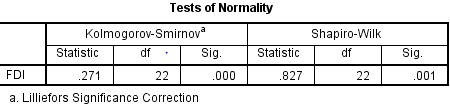# How to test normality statistically?

By Riya Jain & Priya Chetty on March 16, 2020

The previous article explained the importance of testing normality t for a dataset before performing regression. It also explained the various ways to test normality graphically using the SPSS software. However, graphical normality test has several shortcomings, the biggest one being lack of reliability due to the probability of inaccurate results.

For this purpose, statistical or empirical normality tests are conducted. This article explains three such tests using SPSS and E-Views software:

1. Kolmogorov-Smirnov Goodness of Fit (K-S) test,
2. Jarque-Bera test and,
3. Shapiro-Wilk test.

Normal distribution of data is also called ‘Gaussian distribution’. The below equation shows the mathematical formula for normal or gaussian distribution.

## Importance of testing normality of a dataset

Normality tests help in checking whether the data is normally distributed or not. Statistical tests such as regression assume the existence of normally distributed data. For example, simple linear regression analysis for determining the impact of social factors on women’s empowerment does not include the normality test of the dataset. However, this assumption is not always accepted.

Data scientists strictly prefer to test normality and work on normally distributed data because of its benefits (Parbhakar, 2018). Some of the important characteristics of a normal distribution are –

• Provide a high confidence level in the analysis.
• Better model fit for nature and social science-based studies.

Thus, considering the characteristics of normally distributed data, a normality test needs to be performed for generating more effective results.

## Methods to test normality

A normality test is typically represented by the below hypothesis.

H0: Sample is not derived from a normally distributed population.

Ha: Sample is derived from a normally distributed population.

### Statistical tests of checking normality of a dataset

Statistical test of normality calculates the probability of deriving sample from the normally distributed population. The empirical methods of normality test are classified as under.

* Best-suited for the sample between 3 and 2000 but can work till 5000. However, work best for dataset < 50.

Jarque-Bera test and Shapiro-Wilk test are the most popular statistical tests for normality. Shapiro-Wilk test can be performed in SPSS and Stata. EViews and Stata support the Jarque-Bera test. However, K-S Test can only be applied in SPSS.

## Case example of statistical tests of normality

This case example involves the representation of empirical or statistical tests of normality using data of FDI inflows of India from 1994-2015. The results are represented below.

### K-S test and Shapiro-Wilk test of normality in SPSSFigure 1: K-S test and Shapiro Wilk test results of normality in SPSS

The table shows that the significance or p-value of the K-S test (0.000) is less than the tolerable significance level of 5% i.e. 0.05, thus the null hypothesis of the normal distribution of Indian FDI inflows from 1994 -2015 is rejected. Shapiro-Wilk test results are similar to K-S test results i.e. the p-value of 0.001 < 0.05, hence, the null hypothesis is rejected. Hence, the FDI Inflows sample is not derived from the normally distributed population.